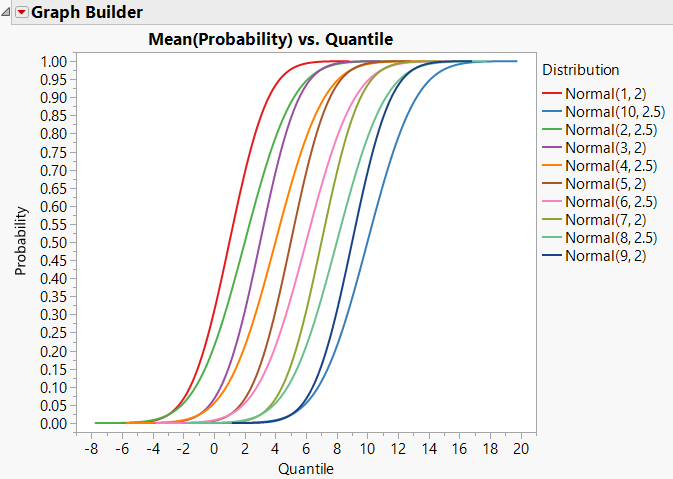Our World Statistics Day conversations have been a great reminder of how much statistics can inform our lives. Do you have an example of how statistics has made a difference in your life? Share your story with the Community!
Choose Language Hide Translation Bar
Highlighted

## How to create an CDF plot in Graph Builder

What would be the best way to create a CDF plot in Graph Builder, comparable to Fit Y by X (CDF / CumProb), but with the functionality of Graph Builder like Overlay, Group, Color etc.?

9 REPLIES 9
Highlighted

## Re: How to create an CDF plot in Graph Builder

Here's an example using repeated sequences of probabilities, and quantiles of those probabilities for different distributions:Here's the script for the table I made to illustrate:

``````New Table( "Untitled",
New Script(
"Normal CDFs",
Graph Builder(
Size( 526, 452 ),
Show Control Panel( 0 ),
Variables( X( :Quantile ), Y( :Probability ), Overlay( :Distribution ) ),
Elements( Line( X, Y, Legend( 18 ) ) )
)
),
New Column( "Probability",
Numeric,
"Continuous",
Format( "Best", 12 ),
Formula( 1 / (1 + Exp( -Sequence( -10, 10, 0.1, 1 ) )) )
),
New Column( "Quantile",
Numeric,
"Continuous",
Format( "Best", 12 ),
Formula( Normal Quantile( :Probability, :mu, :sigma ) )
),
New Column( "mu",
Numeric,
"Continuous",
Format( "Best", 12 ),
Formula( Sequence( 1, 10, 1, 402 ) )
),
New Column( "sigma",
Numeric,
"Continuous",
Format( "Best", 12 ),
Formula( Sequence( 2, 2.5, 0.5, 402 ) )
),
New Column( "Distribution",
Character,
"Nominal",
Formula( "Normal(" || Char( :mu ) || ", " || Char( :sigma ) || ")" ),
Set Selected
)
)``````
-- Cameron Willden
Highlighted

## Re: How to create an CDF plot in Graph Builder

Thanks for fast reply and interesting samples Cameron. What must be done to create a CDF Plot in Graph Builder the attached Data similar to the CDF plot result from the Y bx X platform?

Highlighted

## Re: How to create an CDF plot in Graph Builder

Here's an example with 4 Poisson distributions:

``````New Table( "Poisson CDF Example",
New Script(
"Poisson CDF Comparison",
Graph Builder(
Size( 526, 452 ),
Show Control Panel( 0 ),
Variables( X( :X ), Y( :Probability ), Overlay( :Distribution ) ),
Elements( Line( X, Y, Legend( 19 ), Connection( "Step" ) ) ),
SendToReport(
Dispatch(
{},
"X",
ScaleBox,
{Min( -1 ), Max( 12 ), Inc( 10 ), Minor Ticks( 1 )}
)
)
)
),
New Column( "Distribution",
Character,
"Nominal",
Formula( "Pois(" || Char( :Lambda ) || ")" )
),
New Column( "Lambda",
Numeric,
"Continuous",
Format( "Best", 12 ),
Formula( If( Row() < 51, 0.5, Row() < 101, 2, Row() < 151, 4, 1.25 ) )
),
New Column( "X",
Numeric,
"Continuous",
Format( "Best", 12 ),
Formula( Sequence( -1, 48, 1, 1 ) )
),
New Column( "Probability",
Numeric,
"Continuous",
Format( "Best", 12 ),
Formula( Poisson Distribution( :Lambda, :X ) ),
Set Selected
)
)``````

The key with a step function CDF is to turn off the smoother line in Graph Builder, and connect the points using Line.  Then change Connection in the control panel to "Step".

-- Cameron Willden
Highlighted

## Re: How to create an CDF plot in Graph Builder

Thanks Cameron for providing solutions for the Poisson distribution and the hint for connection the points step wise. I did use the Poisson numbers only to generate the data.

What would be the way for a given data set without knowing how they are distributed to create a CDF plot?

Highlighted

## Re: How to create an CDF plot in Graph Builder

Would you fit a parametric distribution to them or would you use something like Kaplan-Meier to fit the CDF to the data?

Also, if you don’t have tons of data for each group, just putting the fitted CDF values for your data against the actual data values will probably leave some gaps in the plotted CDF. That’s why I created a sequence of values to plot so I make sure I fill in the whole range.

This makes it a 2 step process. 1. fit distributions to each group of data and get the distribution parameter estimates. 2. Create a data table to plot the CDF probabilities (computed with column formulas) against a sequence of values that covers the range.

This is a laborious process, but would be ok if you don’t have tons of groups and only need to do it once. If you need something robust, you probably need a scripted solution

-- Cameron Willden
Highlighted

## Re: How to create an CDF plot in Graph Builder

This is a very nice chart. It would help if you can give the same example using counts and not probability. I suppose calculating the probability is frequency/total? the problem is binning the counts...

Thanks

Highlighted

## Re: How to create an CDF plot in Graph Builder

Use the Distribution platform to get the probabilities. Click the red triangle for the variable in Distribution and select Save > Prob Scores. Now use this new data column in the Y role in Graph Builder and the original data column in the X role.

Learn it once, use it forever!
Highlighted

## Re: How to create an CDF plot in Graph Builder

Thanks for your support. I did try the  Prob Score method. However I got different result for the zero potion of the attached file. The Distribution and the CDF Plot from the Y by X Platform are delivering a zero portion of 60.4 %.

Graph builder and Prob Score are delivering a 30 % for the zero portion.

Highlighted

## Re: How to create an CDF plot in Graph Builder

Sorry. I did not notice the discrepency before. JMP Help says:

For N nonmissing scores, the probability score of a value is computed as the averaged rank of that value divided by N + 1. This column is similar to the empirical cumulative distribution function.

So they are not the same. I can't find a substitute.

Learn it once, use it forever!
Article Labels

There are no labels assigned to this post.